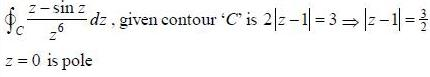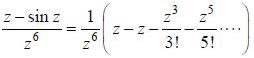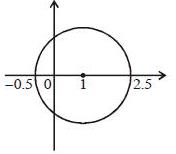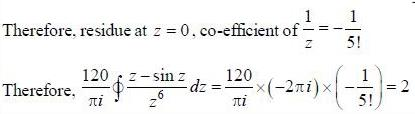Courses

# Test: Mathematical Physics - 2

## 20 Questions MCQ Test GATE Physics Mock Test Series | Test: Mathematical Physics - 2

Description
This mock test of Test: Mathematical Physics - 2 for GATE helps you for every GATE entrance exam. This contains 20 Multiple Choice Questions for GATE Test: Mathematical Physics - 2 (mcq) to study with solutions a complete question bank. The solved questions answers in this Test: Mathematical Physics - 2 quiz give you a good mix of easy questions and tough questions. GATE students definitely take this Test: Mathematical Physics - 2 exercise for a better result in the exam. You can find other Test: Mathematical Physics - 2 extra questions, long questions & short questions for GATE on EduRev as well by searching above.
QUESTION: 1

### For the function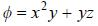at the point (1, 2, -1), find its rate of change with distance in the direction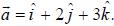Solution: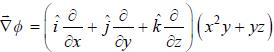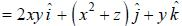Therefore,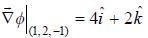Therefore, rate of change with distance in the direction of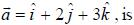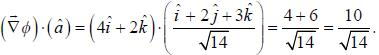QUESTION: 2

### Evaluate the line integral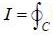(exy + cos x sin y) dx + (ex + sin x cos y)dy, around the curve 'C'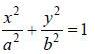Solution: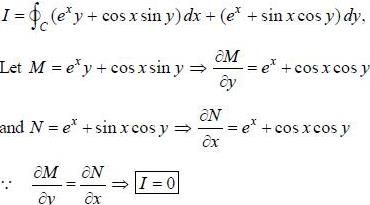Note: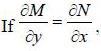. then vector function is conservative and hence line integral (work clone) along a closed path is zero)

QUESTION: 3

### If a unitary matrix U is written as A + iB, where A and B are Hermitian matrix with non degenerate eigenval­ ues. then

Solution:

Since. U is unitary matrix.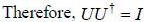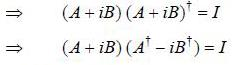or (A + iB) (A - iB) = I  (since, A and B are Hermitian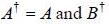= B)
A2 + B2 + i(BA - AB) = I
Now. equating real and imaginary
Part. A2 + B2 = I and BA - AB = 0

QUESTION: 4

If (A)nxn is a square matrix, and elements of matrix A(aij) are such that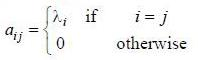then value of det (eA) is equal to

Solution: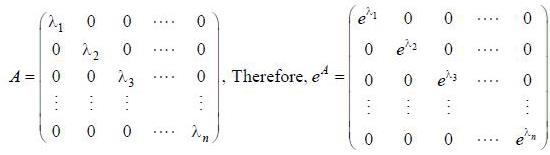We know that, det (eA) = etrace A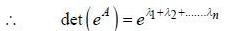*Answer can only contain numeric values
QUESTION: 5

If solution y(x), of the differential equation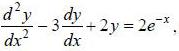subjected to the boundary conditions y(0) = 2, y'(0) = 1 has the form y(x) = Ae-x + Bex + Ce2x, then the value of A + B - C is
(upto two decimal places)

Solution:

D2 - 3D + 2 = 0
(D - 2) (D - 1) = 0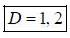Therefore, complementary function Bex + Ce2x and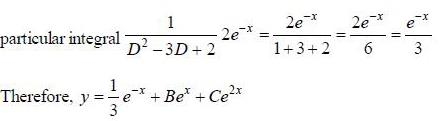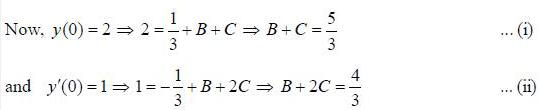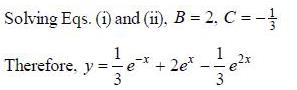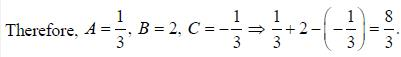*Answer can only contain numeric values
QUESTION: 6

The range of values ofz, for which the following complex power series converges,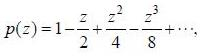is Izl < A, then A is .................. (answer should be an integer)

Solution: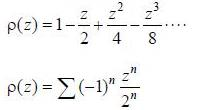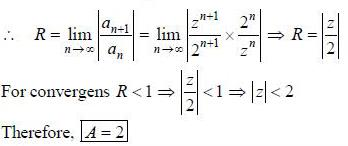QUESTION: 7

If the matrix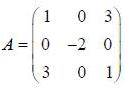can be diagonalised by a transformation of the form S* AS = A', where S has the normalized eigenvectors of A as its columns, then A' is

Solution:

A' will be a diagonal matrix, whose diagonal elements are the eigenvalues of A.
Therefore, to find eigenvalues of A.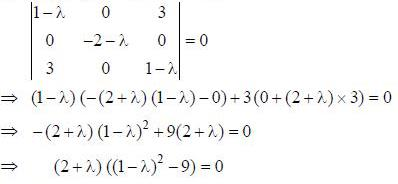Therefore, eigenvalues are λ = - 2, - 2, 4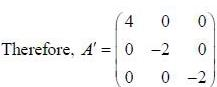QUESTION: 8

If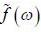is the fourier transformation of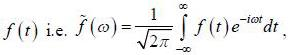then the fourier trans form of f ' (t) is

Solution:

Fourier transfonn of f' (t)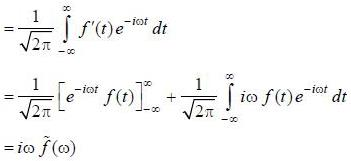QUESTION: 9

If a complex function f (z) lias a pole of order m at z = z0. then f'(z) has a pole of order

Solution: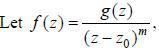where g is analytic at z0 and g(z0) ≠ 0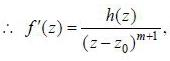where h(z) = (z - z0) g'(z) - mg(z) is analytic at z = z0 and h(z0) = - mg (z0) ≠ 0
∴ f' (z) has a pole of order (m + 1) at z = z0.

QUESTION: 10

The inverse laplace transfonn of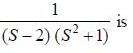Solution: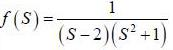Using partial fraction.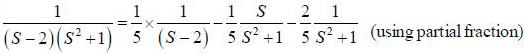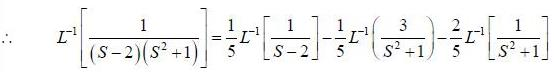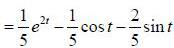*Answer can only contain numeric values
QUESTION: 11

If the fourier series for Isin θ| in the range -π < θ < π is given by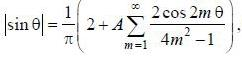then the value of A is .........

Solution: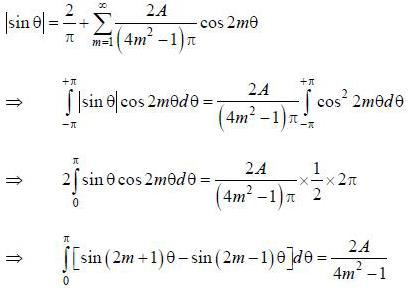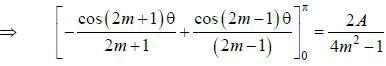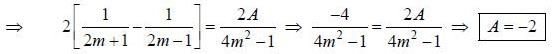*Answer can only contain numeric values
QUESTION: 12

Value of the integral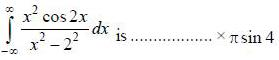Solution: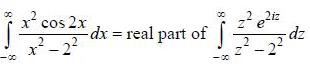Poles are at z = + 2
Therefore, residue at 2 = 2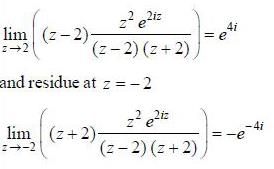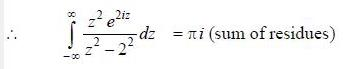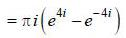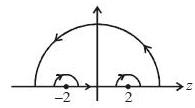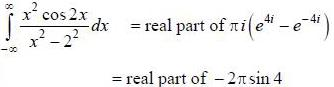*Answer can only contain numeric values
QUESTION: 13

If the fourier expansion of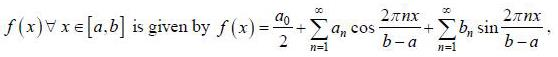then the value of ar if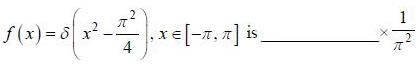Solution: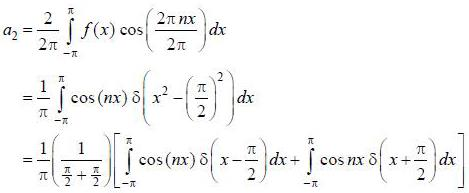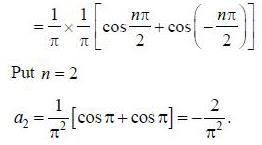QUESTION: 14

If z = xy, where y = tan-1 t and x = sin t, then the value of dz/dt is equal to

Solution: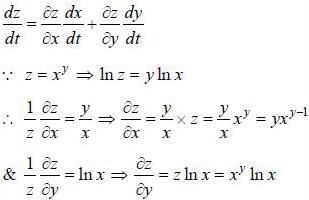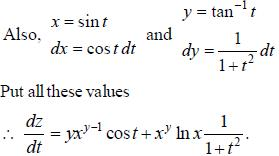QUESTION: 15

If y (x) is the solution of the differential equation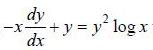with y(1) = -1, then

Solution: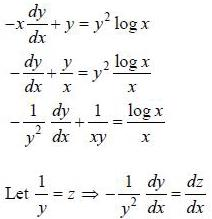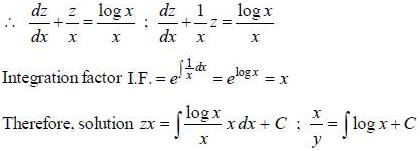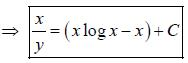Now, y (1) = -1, put x = 1, y = -1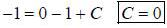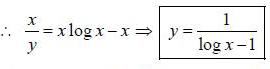∴ for y to be defined log x must be defined i.e.. x > 0
Also when log x - 1 = 0, log x = 1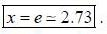So in the range 0 < x < 3 (option (b)). there will be a point x = 2.73 at whichy is not defined.
Also at x = e : y → ∞ (blow up).

QUESTION: 16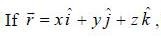then the value of integral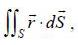where ‘S’ is the surface given by z = 12, x2 - y2 < 25 (taken anticlockwise), is

Solution: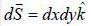∴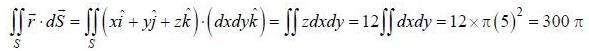QUESTION: 17

The fourier complex transfoem of f(x), where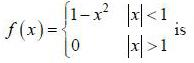Solution:

Fourier transform of f (x)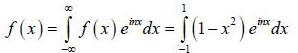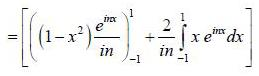(integrating by parts)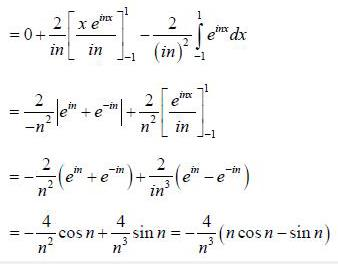QUESTION: 18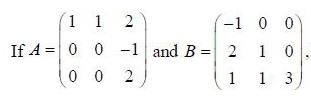then which of the following statements is true about A and B

Solution:

Since A is upper trinagular and B is lower triangular matrix. Hence, their eigenvalues are the principle diagonal elements.
Therefore, eigenvalues of A are 1, 0, 2 and eigenvalues of B are -1,1, 3
Since eigenvalues of A and B are all distinct, hence their corresponding eigenvectors will be linearly independent.
Hence, both A and B are digonalizable.

QUESTION: 19

The Laplace transform of f (t) = t2 cos at is

Solution: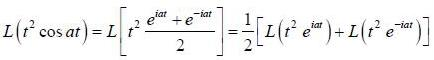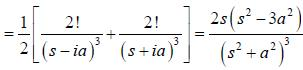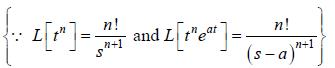*Answer can only contain numeric values
QUESTION: 20

The value of the integral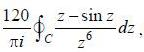where C is closecl contour defined by the equation 2 |z - 1| - 3 = 0, traversed in the clockwise direction, is _______________________ (answer should be an integers)

Solution: